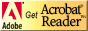## EE 231

Lecture Outline for Spring 2008

1. Wednesday 8/27

• Introduction to Digital Electronics
• Mano, Sections 1.1 through 1.4
• Course Overview
• Conversion between decimal and hexadecimal numbers

2. Friday 8/29

• Mano, Sections 1.5 through 1.7
• One's and two' complements of binary numbers
• Taking one's and two's complements of a binary number using hexadecimal numbers
• Using two's complements to subtract binary and hex numbers
• Unsigned binary numbers
• Two's complement representation of signed binary numbers
• Binary codes

3. Wednesday 9/3

• Register Transfer Logic and Basic Logic Gates
• Introduction to Boolean Algebra
• Mano, Sections 1.8 through 1.9
• Mano, Sections 2.1 through 2.4
• What is a register?
• Intro to register transfer logic
• Basic logic gates: AND, OR, NOT
• Intro to Boolean Algebra
• Boolean postulates and theorems
• Proof of Boolean postulates and theorems using truth tables

4. Friday 9/5

• More on Boolean Logic
• Mano, Sections 2.4 through 2.5
• Boolean algebra postulates and theorems
• Simplifying a logical expression using algebraic manipultion
• Complement of a function using DeMorgan's theorem
5. Monday 9/8

• Canonical and Standard Forms
• Mano, Sections 2.6
• Definition of minterm and maxterm
• Expressing a Boolean function in canonical forms: Sum of minterms or product of maxterms
• Expressing a Boolean function in standard forms: Sum of products or product of sums
• Generating a truth table from a Boolean expression
6. Wednesday 9/10

• Logic Operations, Digital Gates, Integrated Circuits
• Mano, Sections 2.7 through 2.9
• 16 logic operations on two inputs
• Logic gates for two inputs --- AND, NAND, OR, NOR, XOR, XNOR
• Brief discussion of digital IC families
Note: Lecture figures may be presented in Adobe Acrobat format. You need to open those using the Adobe Acrobat Reader program. This is a free program available from Adobe:Bill Rison, <rison@ee.nmt.edu >